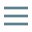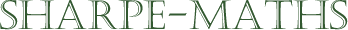Previous topic:
Collecting like terms
Current topic:
Expanding expressions
Next topic:
Multiplying brackets $\big(x \pm a \big)\big(x \pm b \big)$

This section will look at some techniques for expanding and simplifying algebraic expressions. In the first instance, we will be looking at the distributive law, which is defined mathematically as:

$a.(b+c)=a.b+a.c$

We can simply demonstrate that this is true, though proving it is, like so much mathematics, beyond the scope of this section.

$2(5+7) = 2 \times 12= 24$

$2(5+7) = 2 \times 5 + 2 \times 7 = 24$

This will lead us into factorising which is the same process, but backwards. This is what we can an inverse operation.